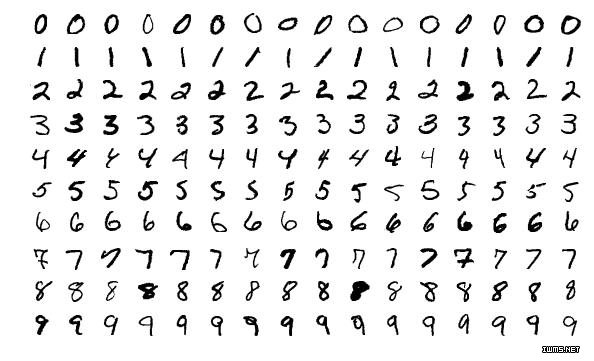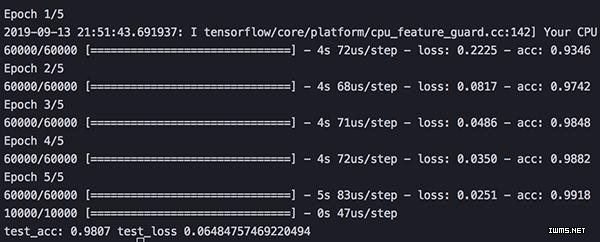﻿ 用Keras编写你的第一个神经网络，用不了30行代码人工智能世界

# 用Keras编写你的第一个神经网络，用不了30行代码

 [日期：2019-10-28] 来源：51CTO  作者：布加迪编译 [字体：大 中 小]

【51CTO.com快译】回想起刚接触AI时，我清楚地记得一些概念看起来有多难。阅读神经网络的简单介绍常常碰到科学论文，里面的公式有你从未见过的符号，但开始编写第一个神经网络实际上容易得多!

HNC Software的联合创始人Robert Hecht-Nielsen博士简单地说。

……神经网络是由许多简单又高度互连的处理单元组成的计算系统，这些处理单元通过对外部输入的动态状态响应来处理信息。

——人工智能专家Maureen Caudill撰写的《神经网络入门：第一部分》，1989年2月MNIST好比是“Hello World”数据集，因为它能够非常简明地演示神经网络的功能。数据集由手写数字组成，我们将训练神经网络识别和分类这些数字。

Keras登场

`pip3 install Keras pip3 install Tensorflow `

`from keras.datasets import mnist from keras import models from keras import layers from keras.utils import to_categorical `

Keras有许多数据集可以用来帮助你学习，对我们来说幸好有MNIST这个数据集。Models和Layers这两个模块可帮助我们构建神经网络，to_categorical用于数据编码，不过稍后有详细介绍。

`(train_images, train_labels), (test_images, test_labels) = mnist.load_data() `

`network = models.Sequential() network.add(layers.Dense(784, activation='relu', input_shape=(28 * 28,))) network.add(layers.Dense(784, activation='relu', input_shape=(28 * 28,)))network.add(layers.Dense(10, activation='softmax'))network.compile(optimizer='adam', loss='categorical_crossentropy', metrics=['accuracy']) `

`network = models.Sequential() `

`network.add(layers.Dense(784, activation='relu', input_shape=(28 * 28,))) network.add(layers.Dense(784, activation='relu', input_shape=(28 * 28,))) network.add(layers.Dense(10, activation='softmax')) `

`network.compile(optimizer='adam', loss='categorical_crossentropy', metrics=['accuracy']) `

`train_images = train_images.reshape((60000, 28 * 28)) train_images = train_images.astype('float32') / 255 test_images = test_images.reshape((10000, 28 * 28)) test_images = test_images.astype('float32') / 255 `

`train_labels = to_categorical(train_labels) test_labels = to_categorical(test_labels) `

`network.fit(train_images, train_labels, epochs=5, batch_size=128) `

`test_loss, test_acc = network.evaluate(test_images, test_labels) print('test_acc:', test_acc, 'test_loss', test_loss) `评论声明 尊重网上道德，遵守中华人民共和国的各项有关法律法规 承担一切因您的行为而直接或间接导致的民事或刑事法律责任 本站管理人员有权保留或删除其管辖留言中的任意内容 本站有权在网站内转载或引用您的评论 参与本评论即表明您已经阅读并接受上述条款
-->